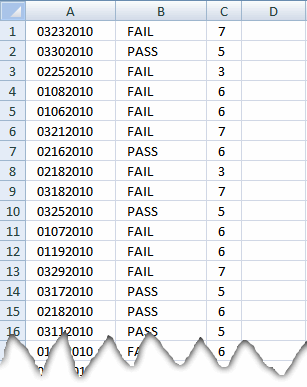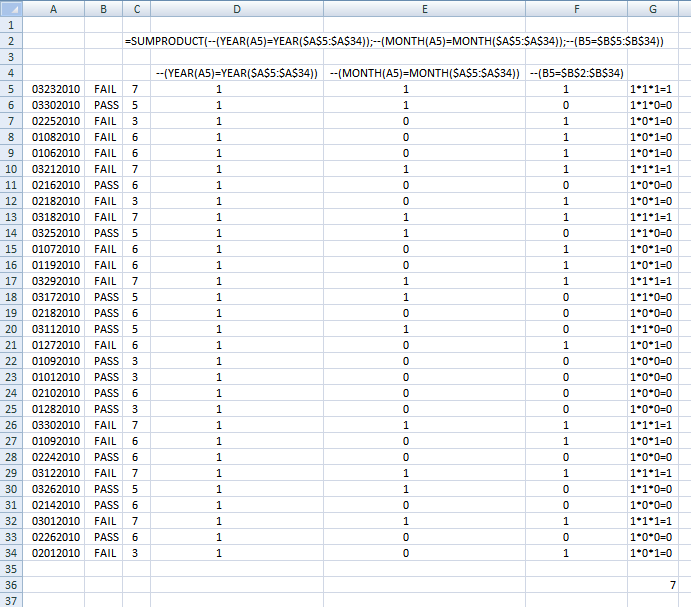Author: Oscar Cronquist Article last updated on January 08, 2019In A column, there are dates in mmddyyy format and in B column, there are two variables uses either "PASS" or "FIAIL". All I want to do is to count the "PASS" in individual month range.

Can someone help me in it. I am able to count days of month from the column A but can not link it with Column B.

Formula in cell C1:

=SUMPRODUCT(--(YEAR(A1)=YEAR(\$A\$1:\$A\$30)), --(MONTH(A1)=MONTH(\$A\$1:\$A\$30)), --(B1=\$B\$1:\$B\$30))

Copy cell C1 and paste down as far as needed.

### Explaining formula in cell C1

#### Step 1 - Create arrays

The YEAR function returns the year from an Excel date.

=SUMPRODUCT(--(YEAR(A1)=YEAR(\$A\$1:\$A\$30)), --(MONTH(A1)=MONTH(\$A\$1:\$A\$30)), --(B1=\$B\$1:\$B\$30))

contains three criteria. The first criterion: --(YEAR(A1)=YEAR(\$A\$1:\$A\$30))

becomes

--(2010=2010,  2010, 2010, 2010, 2010, 2010, 2010, 2010, 2010, 2010, 2010, 2010, 2010, 2010, 2010, 2010, 2010, 2010, 2010, 2010, 2010, 2010, 2010, 2010, 2010, 2010, 2010, 2010, 2010, 2010)

becomes

--(TRUE,  TRUE, TRUE, TRUE, TRUE, TRUE, TRUE, TRUE, TRUE, TRUE, TRUE, TRUE, TRUE, TRUE, TRUE, TRUE, TRUE, TRUE, TRUE, TRUE, TRUE, TRUE, TRUE, TRUE, TRUE, TRUE, TRUE, TRUE, TRUE, TRUE)

and creates this array: (1, 1, 1, 1, 1, 1, 1, 1, 1, 1, 1, 1, 1, 1, 1, 1, 1, 1, 1, 1, 1, 1, 1, 1, 1, 1, 1, 1, 1, 1)

The MONTH function returns a number from 1 to 12 representing the month. Jan = 1, Feb = 2 ... Dec = 12.

The second criterion: --(MONTH(A1)=MONTH(\$A\$1:\$A\$30))

creates this array: (1, 1, 0, 0, 0, 1, 0, 0, 1, 1, 0, 0, 1, 1, 0, 1, 0, 0, 0, 0, 0, 1, 0, 0, 1, 1, 0, 1, 0, 0)

The third criterion: --(B1=\$B\$1:\$B\$30)

creates this array: (1, 0, 1, 1, 1, 1, 0, 1, 1, 0, 1, 1, 1, 0, 0, 0, 1, 0, 0, 0, 0, 1, 1, 0, 1, 0, 0, 1, 0, 1)

#### Step 2 - The product of three arrays

The SUMPRODUCT function multiplies the arrays (product) and then add the numbers in the array and returns a total (sum).

The entire formula now becomes

=SUMPRODUCT((1, 1, 1, 1, 1, 1, 1, 1, 1, 1, 1, 1, 1, 1, 1, 1, 1, 1, 1, 1, 1, 1, 1, 1, 1, 1, 1, 1, 1, 1), (1, 1, 0, 0, 0, 1, 0, 0, 1, 1, 0, 0, 1, 1, 0, 1, 0, 0, 0, 0, 0, 1, 0, 0, 1, 1, 0, 1, 0, 0), (1, 0, 1, 1, 1, 1, 0, 1, 1, 0, 1, 1, 1, 0, 0, 0, 1, 0, 0, 0, 0, 1, 1, 0, 1, 0, 0, 1, 0, 1))

and then

=SUMPRODUCT((1, 1, 1, 1, 1, 1, 1, 1, 1, 1, 1, 1, 1, 1, 1, 1, 1, 1, 1, 1, 1, 1, 1, 1, 1, 1, 1, 1, 1, 1)*(1, 1, 0, 0, 0, 1, 0, 0, 1, 1, 0, 0, 1, 1, 0, 1, 0, 0, 0, 0, 0, 1, 0, 0, 1, 1, 0, 1, 0, 0)*(1, 0, 1, 1, 1, 1, 0, 1, 1, 0, 1, 1, 1, 0, 0, 0, 1, 0, 0, 0, 0, 1, 1, 0, 1, 0, 0, 1, 0, 1))

becomes

#### Step 3 - Sum array

=SUMPRODUCT(1, 0, 0, 0, 0, 1, 0, 0, 1, 0, 0, 0, 1, 0, 0, 0, 0, 0, 0, 0, 0, 1, 0, 0, 1, 0, 0, 1, 0, 0) equals 7.

### Picture of formula calculations in cell C5